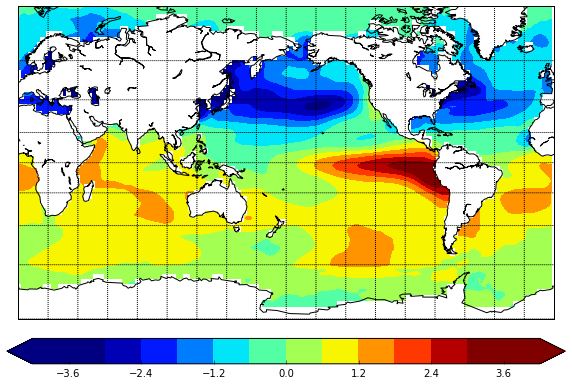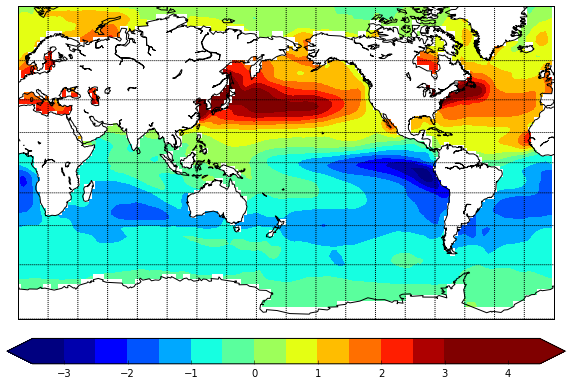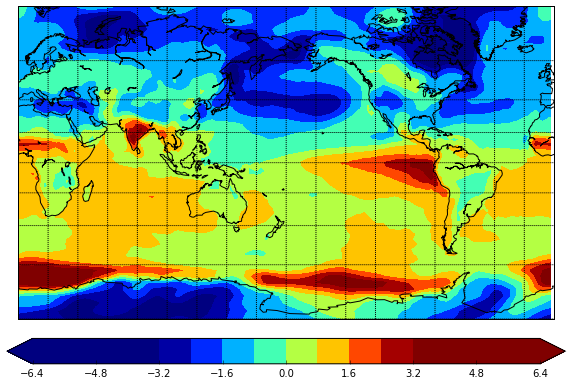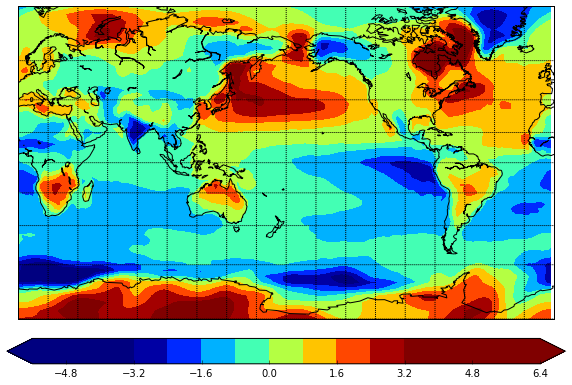# Fisseha Berhane, PhD

#### Data Scientist443-970-2353[email protected] CV Resume### Numpy and Pandas to work with multidimensional arrays¶

The NumPy (Numeric Python) package provides basic routines for manipulating large arrays and matrices of numeric data.

Using Pandas in climate data analysis is a good habit because it provides fast, flexible, and expressive data structures and it is easy and intuitive to apply.

Here, I show the convenience of using numpy together with pandas and matplotlib in working with climate data. I am analysing sea surface temperature and near surface air temperature during the two phases of the ElNino- Southern Oscillation. I show composite analysis based on the sea surface temperature in the Eastern Tropical Pacific SST (5N-5S,150W-90W) from NOAA Climate Prediction Center (CPC).

In :
import pandas as pd
import numpy as np
import matplotlib.pylab as plt
from pandas import Series
from mpl_toolkits.basemap import Basemap
from netCDF4 import Dataset as netcdf       # netcdf4-python module
from netCDF4 import num2date

In :
%matplotlib inline


The Nino 3 index is from NOAA. The air temperature data are NCEP-R1. The sea surface temperature (SST) data can be downloadef from this.

In :
a = netcdf( r'C:\Fish\Python\scripts\sst.mnmean2.v4.nc')
sst = a.variables['SST'][:]
time_sst = a.variables['TIME']
lon_sst = a.variables['LON'][:]
lat_sst = a.variables['LAT'][:]


Similarrly, let's load the air temperature data and get the values of air temperature and the dimensions.

In :
a = netcdf( r'C:\Fish\Python\scripts\air.mon.mean.nc')
air = a.variables['air'][:]
time_air = a.variables['time']
lon_air = a.variables['lon'][:]
lat_air = a.variables['lat'][:]


Let's create variables that contain $datetime$ values using $num2date$ function from $netCDF4$ package.

In :
dates_sst = num2date(time_sst[:], time_sst.units)
dates_air = num2date(time_air[:], time_air.units)


Now, let's convert them to pandas format and then convert timestamps to periods. Both datasets are at monthly resolution.

In :
dates_pd_sst = pd.to_datetime(dates_sst)
dates_pd_air = pd.to_datetime(dates_air)

In :
periods_sst = dates_pd_sst.to_period(freq='M')
periods_air = dates_pd_air.to_period(freq='M')


We can see when the datasets start and end:

In :
periods_sst,periods_air

Out:
(Period('1854-01', 'M'), Period('1948-01', 'M'))
In :
periods_sst[-1],periods_air[-1]

Out:
(Period('2015-05', 'M'), Period('2015-04', 'M'))

Now, let's analyze the period from 1960-2010. Get the time period:

In :
a= (periods_air>=1960)&(periods_air.year<=2010)


Get the air temperature data over the specified period.

In :
air2=air[a,:,:]


Now, let's extract SST over the period we are interested in.

In :
a = (periods_sst.year>=1960)&(periods_sst.year<=2010)
sst2=sst[a,:,:]


See the dimensions of the datasets over the selected period.

In :
sst2.shape, air2.shape

Out:
((612L, 89L, 180L), (612L, 73L, 144L))

The Nino 3 index covers the period from 1950-01 to 2014-12. So, let's create a date range using pandas.

In :
nino=np.loadtxt(r'C:\Fish\Python\scripts\nina3.data')
nino=nino[:,1:]
nino=nino.flatten()
dates = pd.date_range('1950-01', '2015-01', freq='M')
nino = Series(nino, index=dates)


We also need Nino 3 index from 1960 to 2010.

In :
nino=nino['1960':'2010']


Let's standardize the index (subtract the mean and divide by the standard deviation) to use it for composite analysis.

In :
nino=(nino-nino.mean())/nino.std()


Let's check that the index has mean 0 and std of 1

In :
nino.mean(), nino.std()

Out:
(2.9025438552291676e-17, 1.0000000000000002)
In :
nino=np.array(nino)


Get SST during ElNino events.

In :
sst_elnino=sst2[nino>=1.5,:,:]
sst_elnino.shape

Out:
(32L, 89L, 180L)

Get SST during LaNina events.

In :
sst_lanina=sst2[nino<=-1.5,:,:]
sst_lanina.shape

Out:
(46L, 89L, 180L)

The function below is a helper function to plot the composites of SST during ElNino and LaNina.

In :
def sst_composite(sample):
lons, lats = np.meshgrid(lon_sst, lat_sst)

m = Basemap(projection='mill',llcrnrlat=-80,urcrnrlat=80,\
llcrnrlon=0,urcrnrlon=360,lat_ts=20,resolution='c')
x, y = m(lons, lats)
plt.figure(figsize=(10,7))
m.drawcoastlines()
m.drawparallels(np.arange(-80.,81.,20.))
m.drawmeridians(np.arange(-180.,181.,20.))
m.drawmapboundary(fill_color='white')
m.contourf(x,y,(sample.mean(axis=0)-sst2.mean(axis=0)),15,cmap=plt.cm.jet,vmin=-3, vmax=3,extend='both');



Now, let's see the SST pattern during ElNino.

In :
sst_composite(sst_elnino)There is warming in the eastern Pacific Ocean, as expected. We also see posetive PDO like structure and warming over the western Indian Ocean.

Now, let's see the pattern during Lanina.

In :
sst_composite(sst_lanina)In the same fashion, we can plot the composites of near surface temperature and scrutinize the patterns.

In :
air_elnino=air2[nino>=1.5,:,:]
air_elnino.shape

Out:
(32L, 73L, 144L)
In :
air_lanina=air2[nino<=-1.5,:,:]
air_lanina.shape

Out:
(46L, 73L, 144L)

A helper function for the composites:

In :
def air_composite(sample):
lons, lats = np.meshgrid(lon_air, lat_air)

m = Basemap(projection='mill',llcrnrlat=-80,urcrnrlat=80,\
llcrnrlon=0,urcrnrlon=360,lat_ts=20,resolution='c')
x, y = m(lons, lats)
plt.figure(figsize=(10,7))
m.drawcoastlines()
m.drawparallels(np.arange(-80.,81.,20.))
m.drawmeridians(np.arange(-180.,181.,20.))
m.drawmapboundary(fill_color='white')
m.contourf(x,y,(sample.mean(axis=0)-air2.mean(axis=0)),15,cmap=plt.cm.jet,vmin=-3, vmax=3,extend='both');


In :
air_composite(air_elnino)We see that large parts of the northen hemisphere is colder during ElNino.

We can also see the temperature pattern during LaNina.

In :
air_composite(air_lanina)